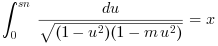Scilab Home page | Wiki | Bug tracker | Forge | Mailing list archives | ATOMS | File exchange
Scilab-Branch-6.1-GIT
Change language to: Français - Português - 日本語 - Русский
Scilab Help >> Special Functions > ellipj

ellipj

Jacobi elliptic functions

Syntax

sn = ellipj(x, m)
[sn, cn] = ellipj(x, m)
[sn, cn, dn] = ellipj(x, m)
struc = ellipj(funames, x, m)

Arguments

x
Array of real or complex numbers inside the fundamental rectangle defined by the elliptic integral.

m
Scalar decimal number in [0,1]: Parameter of the elliptic integral.

sn, cn, dn
Arrays of real or complex numbers, of the size of x: the x-element-wise values of the sn, cn and dn Jacobi elliptic functions.

funames
vector of case-sensitive keywords among "cn", "dn", "sn", "nc", "nd", "ns": Name(s) of Jacobi elliptic functions whose values must be computed and returned.

struc
Structure with fields named with the elements of funames.

Description

If x is real, sn = ellipj(x, m) computes sn such thatThen, if 0 ≤ x ≤ xM where xM is the position of the first sn maximum, we have x==delip(sn, sqrt(m)); if -xM ≤ x ≤ 0, we get x==-delip(-sn, sqrt(m)).

[sn,cn] = ellipj(x,m) and [sn,cn,dn] = ellipj(x,m) additionally compute cn = cos(am) and dn=(1-m.sn2)1/2, where am is the Jacobi elliptic amplitude function as computed with amell().

st = ellipj("dn", x, m) computes values of the dn Jacobi elliptic function for given x values, and returns them in the st.dn field.

Three other nc, nd, ns Jacobi elliptic functions can be computed and returned with this syntax, as nc = 1/cn, nd = 1/dn, and ns = 1/sn.

To compute values of all available Jacobi elliptic functions, we may use st = ellipj(["cn" "nc" "dn" "nd" "sn" "ns"], x, m).

Remark : for complex input numbers x, amell() is used to compute the elliptic amplitude separately of the real and imaginary parts of x. Then, addition formulae are used to compute the complex sn, cn etc [Tee, 1997].The relative numerical accuracy on sn roughly goes as x * [1 to 50] * %eps. Therefore, numerical results for x > 1014 are not relevant. ellipj(%inf, m) is set to NaN.

Examples

With some real input numbers:

sn = ellipj(0:0.5:2, 0.4)
delip(sn, sqrt(0.4))

[sn, cn, dn] = ellipj(-1:0.5:1.5, 0.4)
--> sn = ellipj(0:0.5:2, 0.4)
sn  =
0.   0.4724822   0.8109471   0.9767495   0.9850902

--> delip(sn, sqrt(0.4))
ans  =
0.   0.5   1.   1.5   1.5550387

--> [sn, cn, dn] = ellipj(-1:0.5:1.5, 0.4)
sn  =
-0.8109471  -0.4724822   0.   0.4724822   0.8109471   0.9767495

cn  =
0.5851194   0.8813402   1.   0.8813402   0.5851194   0.2143837

dn  =
0.8584555   0.9543082   1.   0.9543082   0.8584555   0.786374

// Input data
x = linspace(-6,6,300)';
m = [0.2 0.4 0.6 0.8 0.9];
f = ["sn" "dn" "cn" "nd"];

// Computation
st = struct();
for i = 1:length(m)
st(i) = ellipj(f,x,m(i));
end

// Plot
clf
for i = 1:size(f,"*")
subplot(2,2,i)
y = matrix(list2vec(st(f(i))), -1, length(m));
plot2d(x, y,[1 14 28 18 6])
title(f(i), "fontsize",4)
xgrid(color("grey75"),1,7)
end

legend(gca(), msprintf("m =%4.1f\n",m'),1, %f);
lg = gce();
lg.legend_location = "by_coordinates";
lg.position = [-0.095 -0.08];With some complex input numbers:

x = linspace(-6,6,300)';
m = 0.1:0.1:0.9;
sn = [];
for i = 1:length(m)
sn(:,i) = ellipj(x+1.4*%i, m(i));
end

clf
plot2d(imag(sn), real(sn))
title("sn(-6:6 + 1.4i)", "fontsize",4)
xlabel("imag(sn)", "fontsize",2);
ylabel("real(sn)", "fontsize",2);
colordef(gcf(),"none")
gca().data_bounds(1:2) = [-5 5];
gca().tight_limits(1) = "on";
isoview
legend(gca(), msprintf("m =%4.1f\n",m'),"out_upper_right");• amell — Jacobi's am function
• delip — complete and incomplete elliptic integral of first kind
• %k — Jacobi's complete elliptic integral of the first kind (vectorized)
• Tee, Garry J.: Continuous branches of inverses of the 12 Jacobi elliptic functions for real argument”. 1997

History

 Version Description 6.1.0 ellipj() introduced.